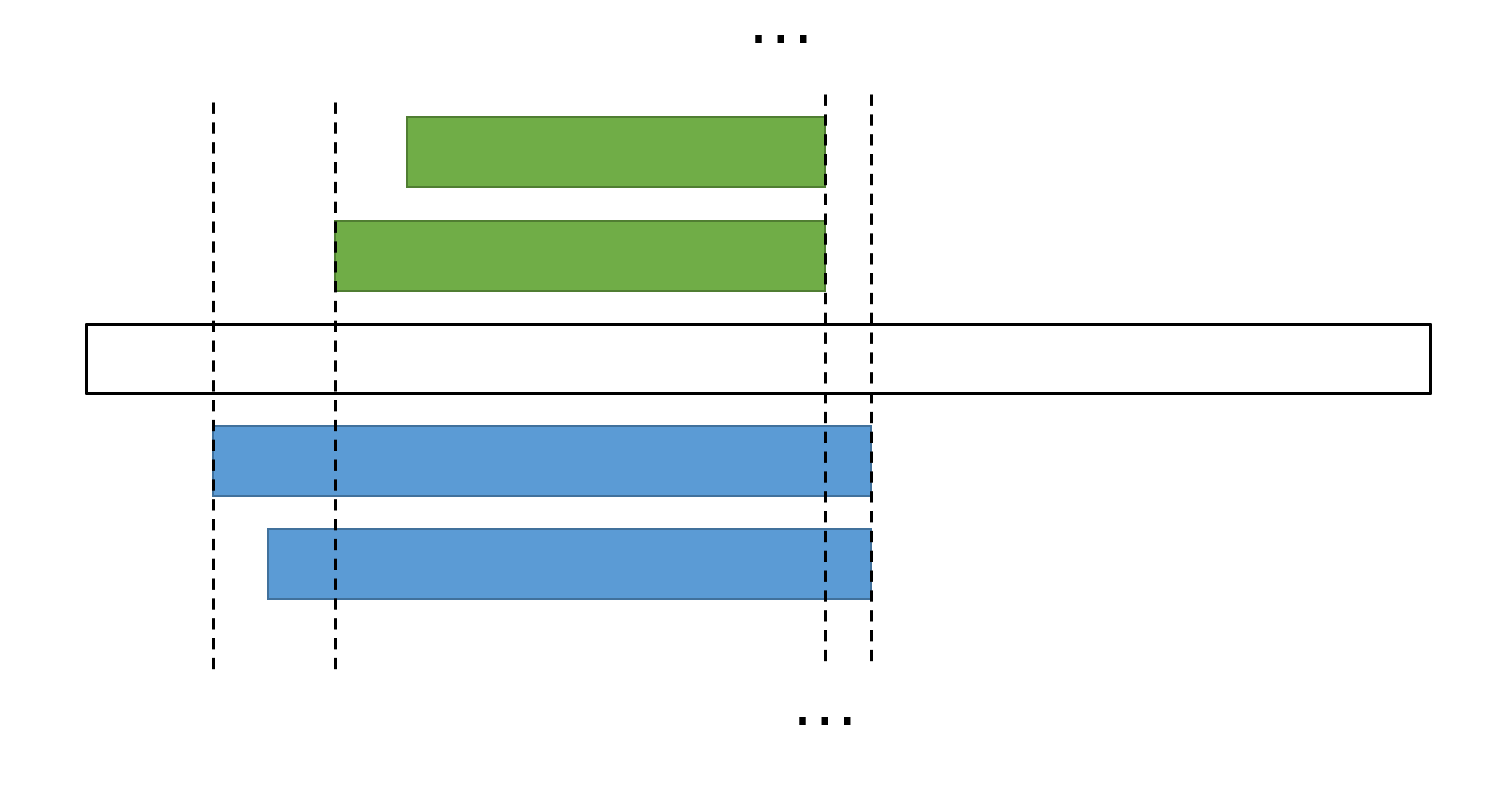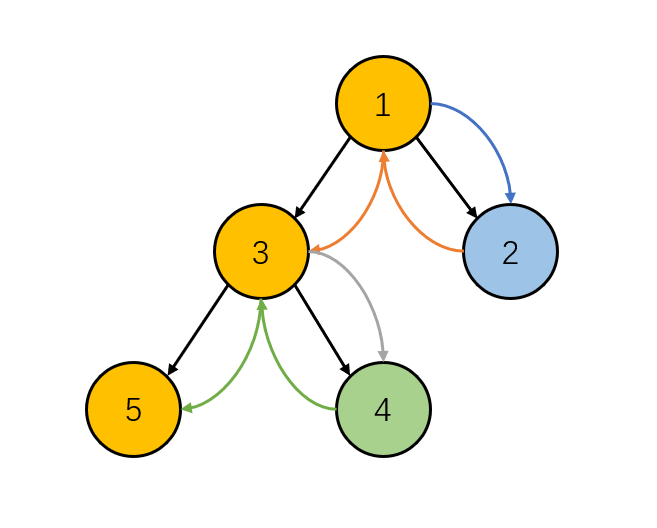# 跃迁引擎启动

cac / commander.js 提供了一个简单直接的 API 来创建命令行应用，你只需要指定用户应该如何使用你的程序参数，就能生成出一个相应的 CLI 应用，包含参数传递，选项解析，版本和帮助命令的自动生成等等。

• 添加了 TypeScript 类型推导
• 移除了部分类型不友好的参数解析
• 添加了多层 sub-commands 的实现

## 题解

KMP 模板题

1. 读入模板串 $S$, 计算出长度为 $\lfloor \sqrt{n} \rfloor$ 的前缀 hash 值和完整串的 hash 值, 时间复杂度 $O(|S|)$, 空间复杂度 $O(1)$;
2. 读入询问串 $T$, 维护 $\lfloor \sqrt{n} \rfloor$ 大小的滑动窗口, 可以求出询问串中所有和这个根号前缀匹配的位置. 相当于, 筛出去了一些必不可能匹配上的位置, 留下来一些位置需要求出相应的全长度的 hash 值.
• 注意到, 直到这里实际上还没有本质的改善, 一是匹配上的位置仍然很多, 二是难以搞出相应的全串 hash 值.
• 根据 (Weak) Periodicity Lemm, 我们可以把匹配上的位置分成至多 $\lceil \sqrt{n} \rceil$ 组等差数列. 具体的, 每组中所有匹配的子串是一个顺次有重叠的列表, 相邻出现位置的距离差相等 (也就是所谓的构成等差数列, 进一步, 重叠部分其实就是根号前缀的 border). 于是, 记录匹配位置的空间被压缩到了 $O(\lfloor \sqrt{n} \rfloor)$.
• 下一个问题是, 我们不仅需要记录匹配的位置, 还需要记录匹配处的前缀 hash 值, 等到处理到该次可能匹配的结束位置时来进行全串的比对. 同样根据上述引理的结论, 同一组等差数列内部, 相邻 2 个匹配位置之间的 hash 值是固定的, 我们只需要记录等差数列的起点, 公差, 终点, 起点的 hash 值, 公差对应字符串部分的 hash 值, 就能表示出这一个等差数列的所有位置信息和 hash 值信息.

# 题解

XLex 正则表达式

rank solved A B C D E F G H I J K L M
96 9 Ø . O Ø Ø O O . O . O . O

# F Examrank solved A B C D E F G H I J K
7 6 O . . O O O O . . . O

# 分析